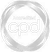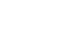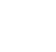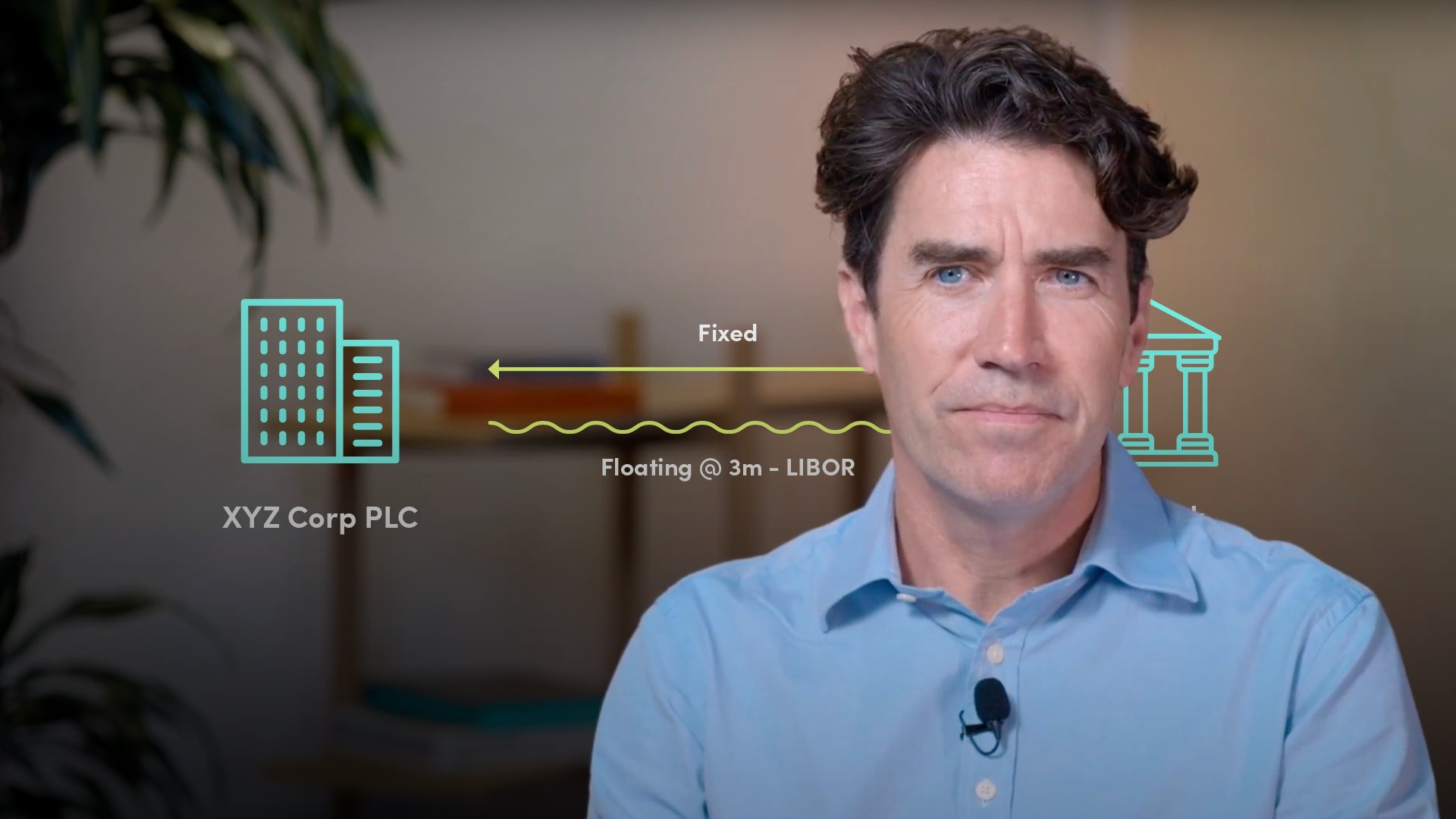# Interest Rate Swaps Pricing### David Leeming

One of the most common types of derivative is an Interest Rate Swap. In this video, David explains how to price Interest Rate Swaps, and introduces the yield curve and the concept of basis swaps.

Derivatives

Corporate DerivativesOne of the most common types of derivative is an Interest Rate Swap. In this video, David explains how to price Interest Rate Swaps, and introduces the yield curve and the concept of basis swaps.

Speak to an expert

Speak to an expert today to access this and all of the content on our platform.### Interest Rate Swaps Pricing

13 mins 42 secs

Overview

Interest rate swaps are when future interest payments are exchanged between two parties. They are contractually obliged to do so.

Key learning objectives:

• Define the A/360 convention, and explain how it affects the amount of money you receive.

• Understand how to calculate interest rate futures.

• Learn the calculation of the NPV of a fixed leg swap

## Speak to an expert

Speak to an expert today to access this and all of the content on our platform.

Summary

#### What is Present Value and how do we calculate its value?

For example, a future cash flow:

The present value is the value of the cash flow discounted by the appropriate interest rate such that if it was invested today, at that interest rate, it will be worth exactly its future value on that day in the future.

I.e. What is the present value of 100 Euros (future) if interest rates for 1 year are 5%? In other words, what do we need to deposit to get 100 euros in 1 years’ time?

The answer: The present value of 100 Euros in a years’ time = 100/1.05 (95.24 Euros)

#### What is a Discount Factor?

These are simply 1 divided by the number used to calculate the future value. Hence, 1/1.05 = 0.9524.

This figure can then be multiplied by all of the future values.

#### How do we calculate Net Present Values for a fixed leg swap?

If you are receiving a fixed leg, the net present value of the swap is the present value of all the received cash flows LESS the present value of all of the floating cash flows.

In order for the swap to be fair to both parties, the Net Present Value of the swap at inception must be equal to zero (or very close to it).

#### How is the A/360 convention used to calculate 3-month SPOT rates?

The Actual 360 assumers there are 360 days a year and you get interest on every single day that you have deposited the cash.

I.e. if you deposited 100 Euros on the 4th of May and 3-month spot rates were 5%, how much will we get back?

The maturity date is 6 August, 92 days. Hence, 100 x (1+(0.05 x 92/360)) = 101.28 Euros. That is to say 1.28 Euros in interest and the initial 100 Euros back.

#### How do we calculate interest rate futures?

Interest rate futures are traded as 100-rate, so a price quoted as 95.00 implies an annual rate of 5% for the period of the future.

#### What are the standardised IMM dates?

These are the 3rd Wednesday of: March, June, September and December.

#### How can we calculate the interest received in 1 years’ time for an Annual vs 3-month swap?

Assume the following:

We deposit 100 Euros on Monday 17th September, and we have interest rates in the future of:

• Sep - 2.7%
• Dec - 3.2%
• Mar – 3.6%
• Jun – 3.9%

Assuming four 90-day periods:

1. In September: 100 x (1+ 0.027 x 90/360)) = 100.675
2. December: 100 x (1 + 0.032 x 90/360)) = 101.4804
3. The same calculations for March and June give us a figure of 3.392%.

## Speak to an expert

Speak to an expert today to access this and all of the content on our platform.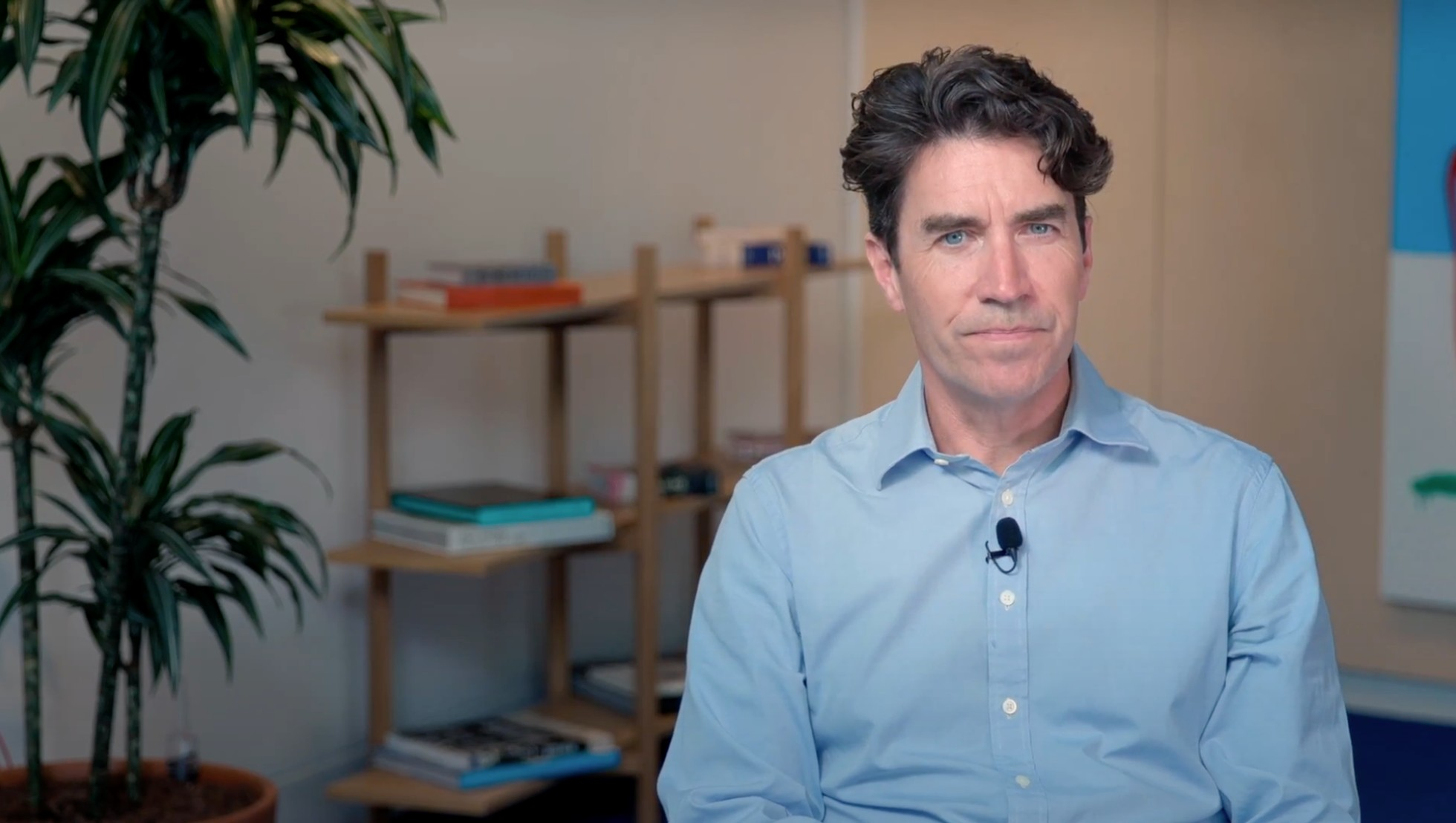### David Leeming

David has over 20 years trading interest rate products. Starting with Italian and Spanish markets in the 90’s enabled him to move to Brazil and then onto Hong Kong. Returning to the UK he ran the GBP Rates Desk at UBS in London trading IRS and Swaptions. He also served on the ISDA Rates Steering Committee and London Clearing Houses’ Default Management Committee.

There are no available videos from "David Leeming"

### Accredited by